# 麒麟子Cocos Creator实用技巧九：棋牌UI全分辨率适配方案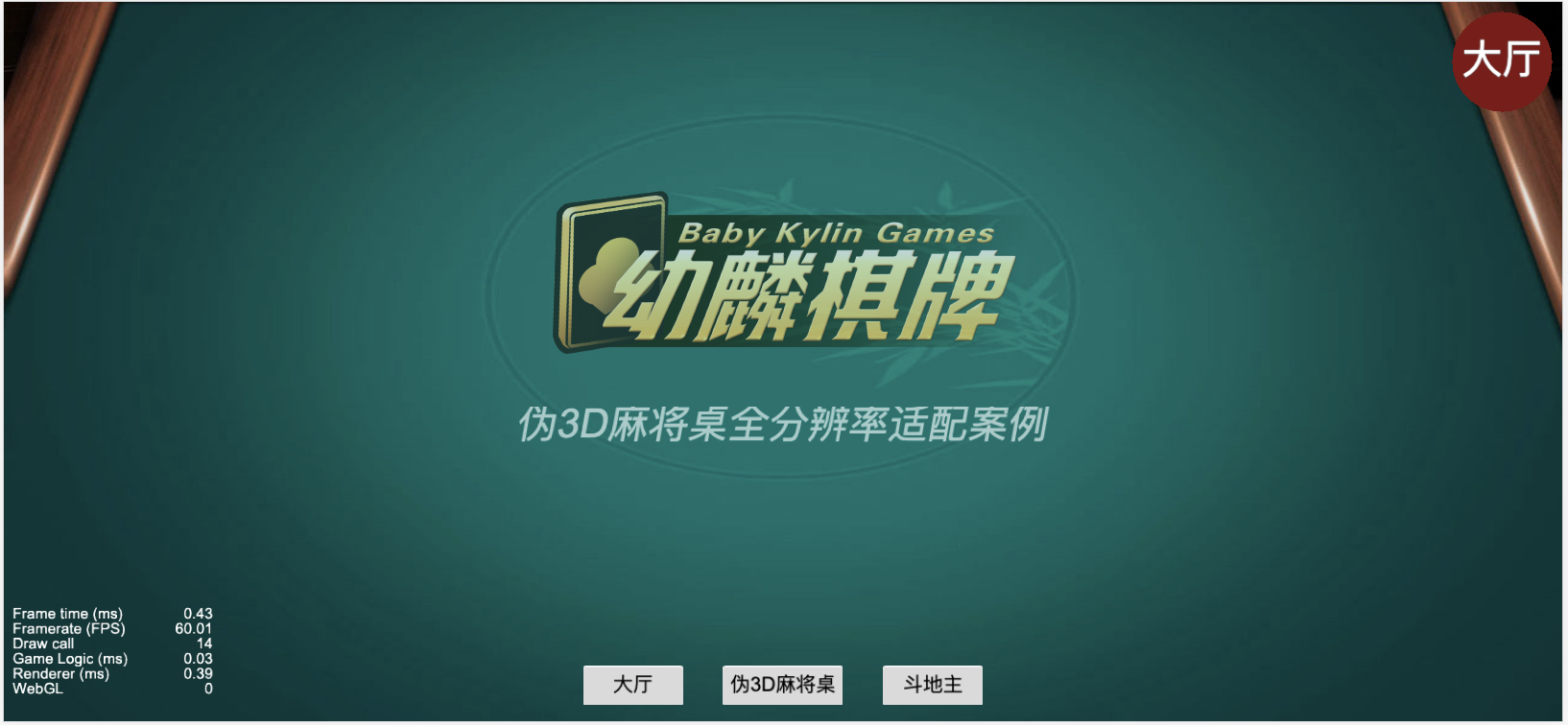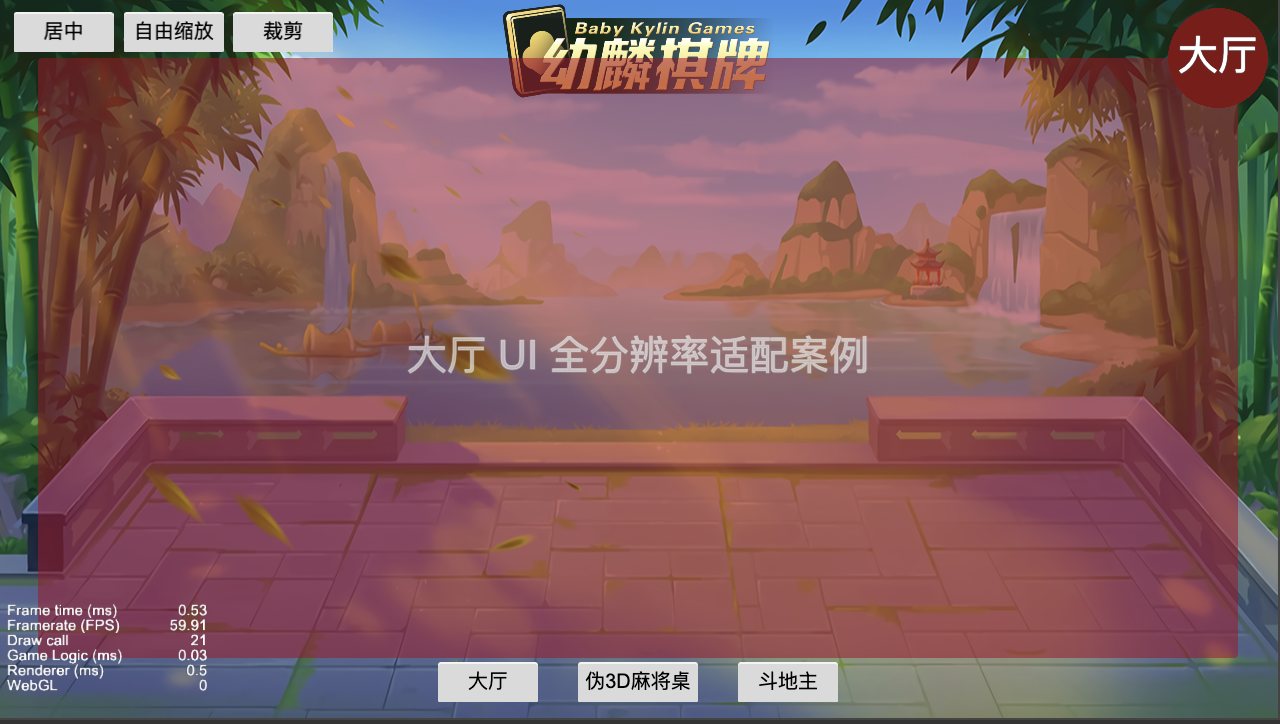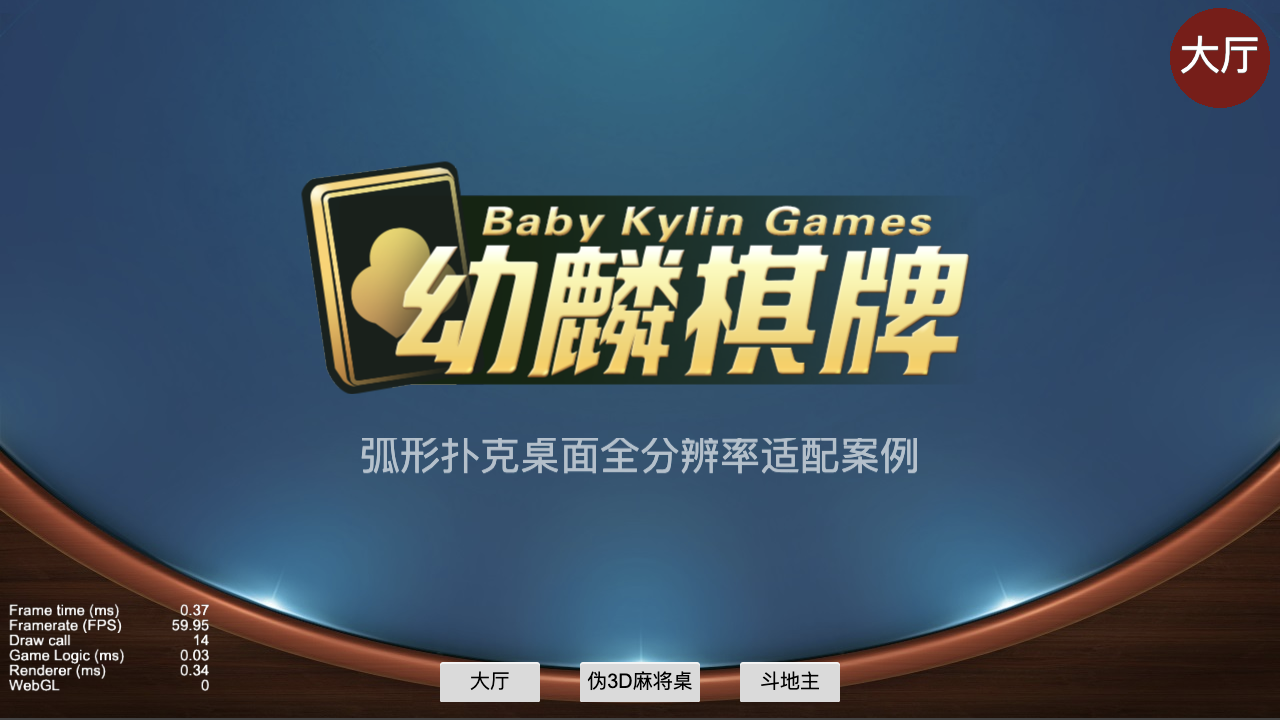PS：黑莓那种1:1也可以的，只是我人为的把它忽略了。

### 一、不同适配策略的表现效果

Cocos Creator的Canvas提供了fitWidth和fitHeight两个开关，可以实现4种效果

1、fitWidth = false, fitHeight = false

对应的是自动选择定宽还是定高，当用户手机比设计分辨率更宽时，使用定宽。 当用户手机比设计分辨率更短时，使用定高。 带来的就是裁剪效果

2、fitWidth = true, fitHeight = false

对应的是定宽效果

3、fitHeight = false,fitHeight = true

4、fitWidth = true,fitHeight = true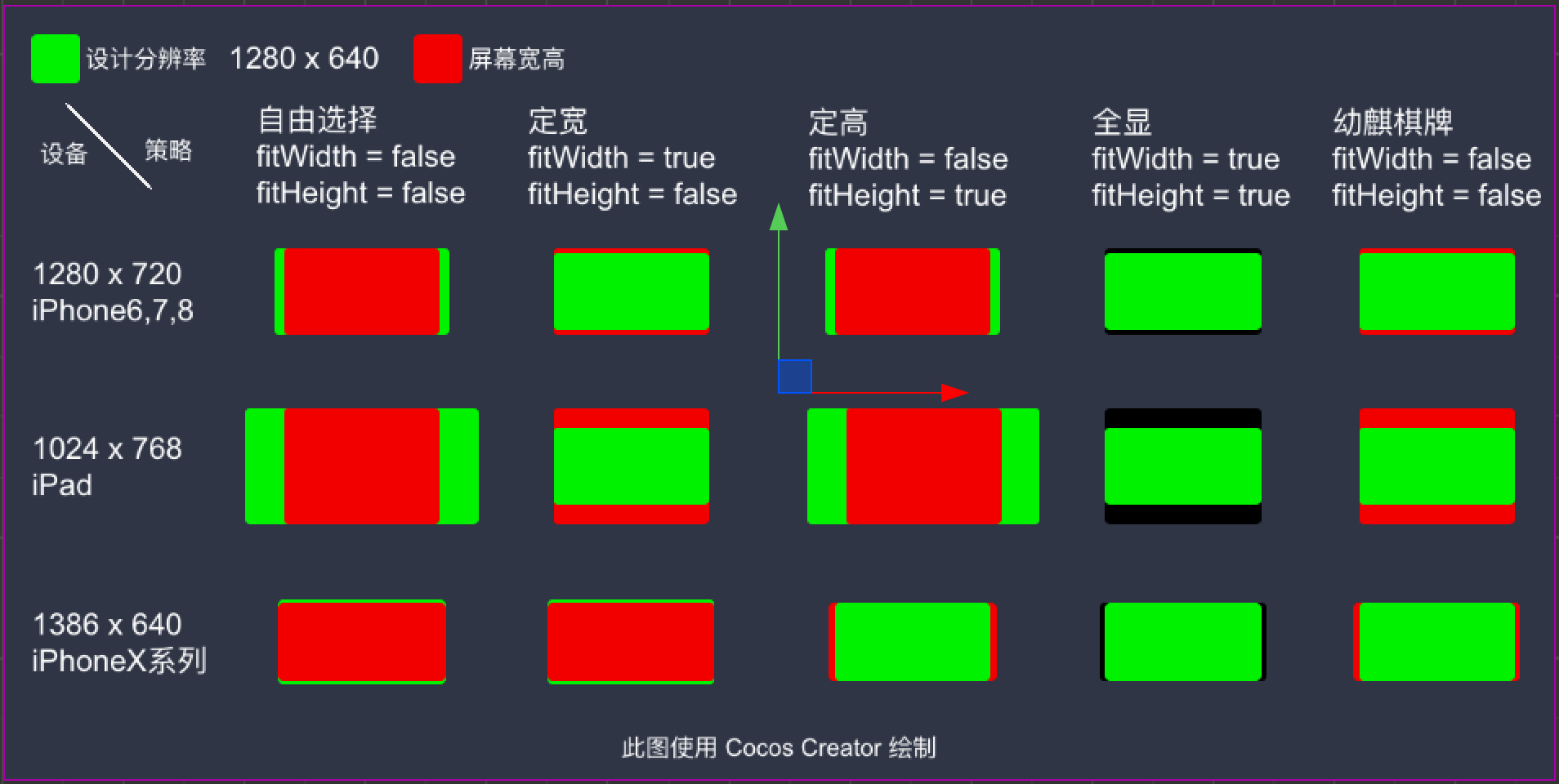### 二、幼麟棋牌的适配模式

1、适配效果介绍

2、全分辨率适配代码实现

    public static resize() {

var cvs = cc.find('Canvas').getComponent(cc.Canvas);
//保存原始设计分辨率，供屏幕大小变化时使用
if(!this.curDR){
this.curDR = cvs.designResolution;
}
var dr = this.curDR;
var s = cc.view.getFrameSize();
var rw = s.width;
var rh = s.height;
var finalW = rw;
var finalH = rh;

if((rw/rh) > (dr.width / dr.height)){
//!#zh: 是否优先将设计分辨率高度撑满视图高度。 */
//cvs.fitHeight = true;

//如果更长，则用定高
finalH = dr.height;
finalW = finalH * rw/rh;
}
else{
/*!#zh: 是否优先将设计分辨率宽度撑满视图宽度。 */
//cvs.fitWidth = true;
//如果更短，则用定宽
finalW = dr.width;
finalH = rh/rw * finalW;
}
cvs.designResolution = cc.size(finalW, finalH);
cvs.node.width = finalW;
cvs.node.height = finalH;
cvs.node.emit('resize');
}

### 三、背景适配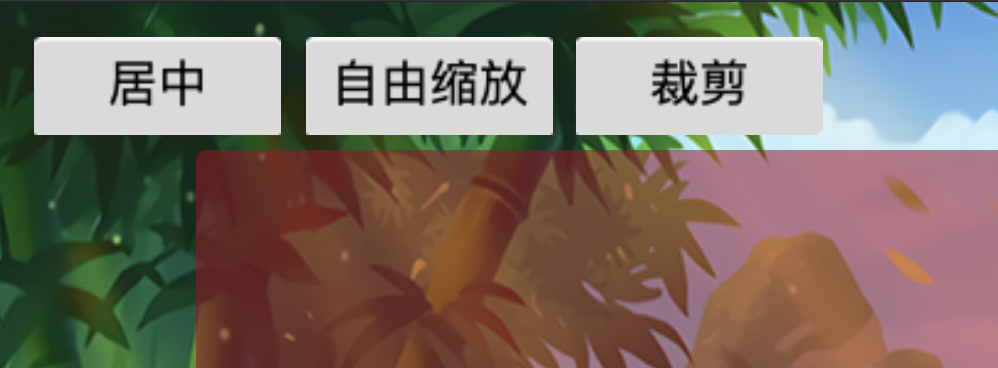resize () {

this.node.width = this.originWidth;
this.node.height = this.originHeight;

//0、居中（居中其实不需要挂这个脚本，浪费效率）
//1、宽高都根据高度拉伸
//2、长边充满
var cvs = cc.find('Canvas').getComponent(cc.Canvas);
var size = cc.view.getFrameSize();

var dr = Utils.curDR;
var scaleMethod = this.mode;

//
var fitWidth = true;
//如果更宽，则使用定高
if((size.width/size.height) > (dr.width / dr.height)){
fitWidth = false;
}

//自由缩放撑满
if(scaleMethod == 1){
if(fitWidth){
this.node.height = this.node.width/size.width * size.height;
}
else{
this.node.width = this.node.height/size.height * size.width;
}
}
//保持等比缩放撑满
else if(scaleMethod == 2){
if(fitWidth){
//定宽表示设备更高了，则以高的缩放为准
var oldHeight = this.node.height;
this.node.height = this.node.width/size.width * size.height;
var scale = this.node.height/oldHeight;
this.node.width = scale * this.node.width;
}
else{
//定高表示设备更宽的，以宽的缩放为准
var oldWidth = this.node.width;
this.node.width = this.node.height/size.height * size.width;
var scale = this.node.width / oldWidth;
this.node.height = scale * this.node.height;
}
}
else{
//默认处理，有黑边
}
}

### 四、麻将桌子适配

    start () {
cc.find('Canvas').on('resize',this.resize.bind(this));
this.resize();
}

resize(){
var canvas = cc.find('Canvas');
if(canvas.width > Utils.curDR.width){
this.node.width = canvas.width;
}
}

### 六、总结

©️2019 CSDN 皮肤主题: Age of Ai 设计师: meimeiellie